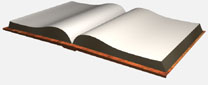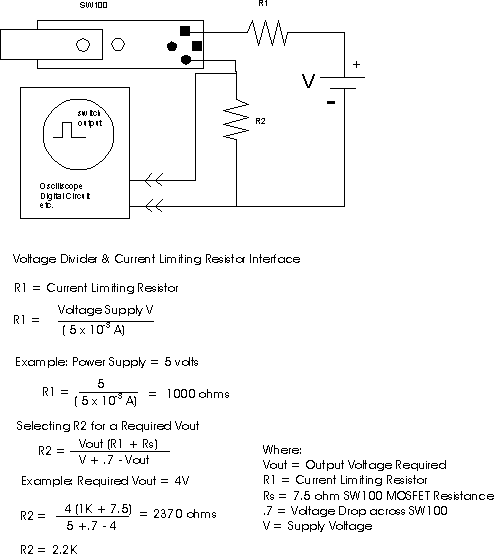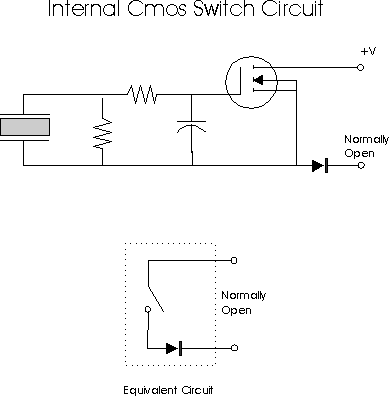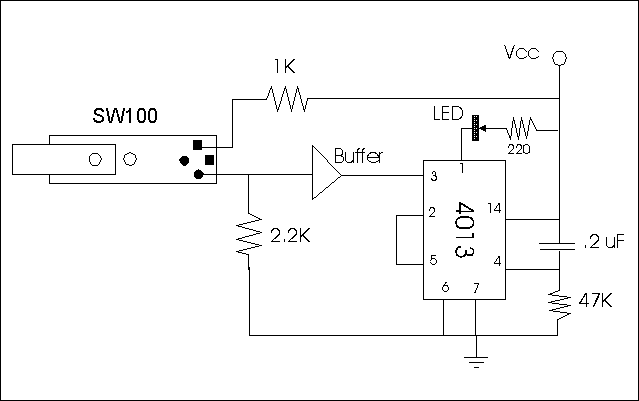# Using the CMOS Switch

How to use the PZ-05 (SW-100) switch is shown in Figure 14. The switch is designed to be used in a variety of applications. Maximum switch voltage is 50 volts and maximum current is 70 mA. Two resistiors R1 and R2 control current and the voltage output pulse (Vout).Figure 14

R1 is a current limiting resistor and R2 adjusts the output voltage. R1 and R2 values may be varied for particular applications using the formulas on Figure 14. In the example we use a digital supply voltage of +5 volts. R1 has a value of 1K. To produce a Vout pulse of +4 volts R2 has a nominal resistance of 2.2K.Figure 15

Figure 15 Illustrates the internal circuitry and equivalent circuit of the piezo switch. Although digital, the PZ-05 piezo switch will not operate the 4013 flip flop circuit in Figure 12 directly. The reason is that the rise and fall times of the digital pulse from the switch is too slow. To solve this problem a buffer (one gate off a non-inverting 4050) is placed between the PZ-05 and the 4013 flip flopFigure 16